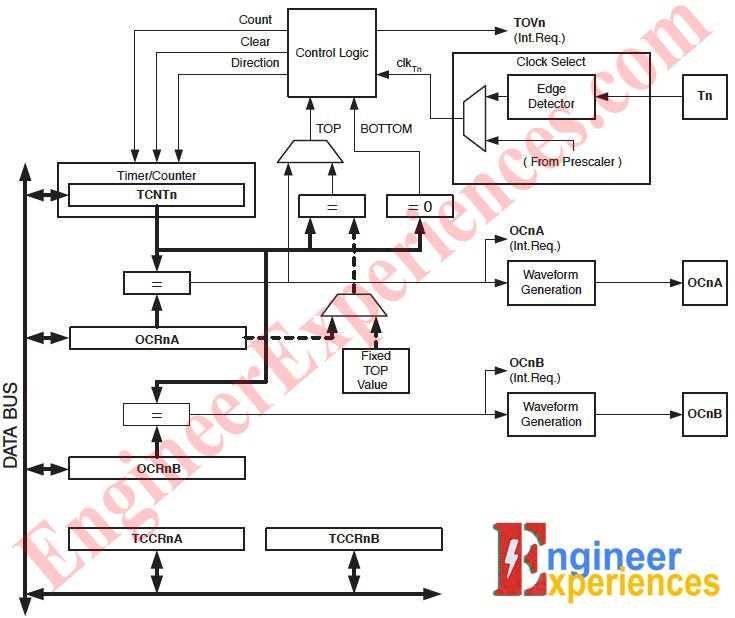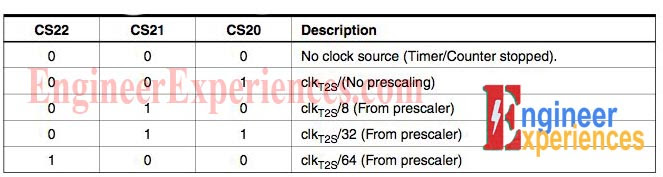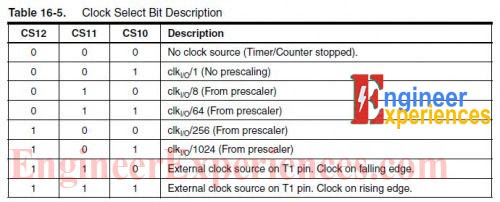# ARDUINO UNO TIMER AND INTERRUPTS

Arduino indeed is a magical thing for a lot of people owing to the amazing and extraordinary thing this little board can do. Just when we believe we have figured all of its secrets we discover something new and today we are going to learn Arduino Uno Timer Interrupts.

The Timer is nothing but a simple clock and like a clock it measures time intervals. A Timer contains a counter which is incremented (or say counts) at certain speed (frequency). The numbers on electronic devices like 16MHz or 2.3GHz all define the clock speed. What that speed signifies is the time required by counter to make a count for e.g., 16MHz (Arduino clock speed) take 1/16000000 sec i.e., 62ns to make a single count.

In Uno there are 3 timers named Timer0, Timer1 and Timer2. These timers have following functions:-

• Timer0- For Uno functions like delay(), millis(), micros() or delaymicros().
• Timer1- For the working of servo library.
• Timer2- For functions like tone(), notone().

Along with these functions these 3 timers are also responsible for generating the PWM Output when analogWrite() command is used in the PMW designated pin.

Timer2, Timer0 are 8-bit timer i.e., they can store maximum count value of 2^8=256 and then restart from zero while Timer1 is a 16-bit timer and can store maximum count value of 2^16=65536 and then restart.

Fig.1 shows schematic of an 8-bit Uno timer. Various registers (memory units!!) are arranged in particular fashion that makes up a timer. The values stored in this registers makes a timer to work in a certain manner and if we change this values we can bend the timer working according to our needs.Figure 1: Schematic of 8 bit arduino UNO Timer.

## IMPORTANT REGISTERS OF A TIMER:

Before moving to configure the timer let’s look at some of the important registers of a timer-

TCCRnA/B- Stands for Timer/Counter Control Registers.

• Holds the main control bits of the timer.
• Control the mode of timer using the WGM bits.
• Control the prescalers of timer.

A prescaler allow us to setup the clock speed of the timer we are working on. Uno supports prescalers as 1, 8,64,256,1024. Consider prescaler as a constant with which if we divide the Primary clock frequency (i.e., 16MHz) we get our timer working frequency. For example we want the speed of clock to be slowed down and we setup the value of prescaler as 8 then the frequency of our timer would slow down to 16MHz/8 = 2MHz i.e., instead of having counter increment at 62ns now we will have it at 500ns.

TCNTn- Stands for Timer/Counter Register.

• Control the counter value (Number of counts).

OCRnA/B- Stands for Output Compare Register.

• As the name signifies, values in these register are used to compare the counter value for some actions.

TIMSKn- Stands for Timer/Counter Mask In Registers.

• They help in actuating certain timer functions.

Setting these registers can allow us to perform several operations and one such thing is “Interrupts” or “Timer Interrupts”. An Interrupt is a set of command that is executed interrupting the source code instructions. We all know Uno execute instruction step by step but what if we want some command to be executed on a regular basis and while doing so the original code should stop at its current position that is when interrupt comes in play. An interrupt allows:-

• Executing a command i.e., receive input or deliver output at fixed intervals.
• Modify the PWM output at PWM pins.
• Generate waveforms of certain frequencies.

To setup an Interrupt we have to configure the timer. Various modes are present to configure the timers of Uno-

• Normal Mode
• CTC Mode
• PWM Mode

We’ll use the most commonly used mode to configure the timer and setup interrupts, the CTC Mode.

CTC stands for Clear Timer on Compare match. Working of CTC is simple- Define a counter value in a register (OCRnA), setup the speed of the timer clock, now when the timer counts up to the compare register value the interrupt takes place and the timer gets restarted once again.

Using this we can execute a command at regular basis at fixed intervals.

Consider we want Interrupt at a frequency of f Hz.

The Counter Compare Register value is given by – [16000000/ (f*Prescaler)]-1.( -1 account as registers are indexed from 0.  )

It should be noted that if the timer used are timer0 and timer2 then this counter value should be less than 256 as they are 8-bit timers and in case of timer1 it should be less than 65536. To reduce the value you can select the large value of the prescaler (from 1, 8, 64, 256, and 1024).

## Example:

Let’s consider a code required to setup an interrupt at 2 KHz in timer0 –

```Prescaler= 64, Compare value-[16000000/2000*64]-1= 124 (124<256)

void setup() {```
1. `cli(); // disable interrupt`
2. `TCCR0A = 0;`
3. `TCCROB = 0;`
4. `TCNT0 = 0; // initialize the counter from 0`
5. `OCR0A = 124; // sets the counter compare value`
6. `TCCR0A |= (1<<WGM01); // enable the CTC mode`
7. `TCCR0B |= (1<<CS01) (1<<CS00); sets the control scale bits for the timer`
8. `TIMSK0 |= (1<<OCIE0A); //enable the interrupt`
9. `sei(); //allows interrupts`
`}`

Now let’s look at the code-

1, 2, 3, 4- are simple as we are just initializing the timer.

5- Recall the OCRnA was a Output Compare Register. So we store the Compare Counter value in this register.

6- These WGM value can are obtained from the datasheet of Atmega 328. Summarized as-

```TCCR0A |= (1<<WGM01); //timer0

TCCR1B |= (1<<WGM12); //timer1

TCCR2A |= (1<<WGM21); //timer2```

7- The CS values are obtained from the table 2, table 3, table 1 according to the prescaler selected and the respective timer.TABLE 1: Arduino UNO timer and interruptsTABLE 2: Arduino Timer and Interrupts, Clock select bit description.Table 3: Arduino interrupts and timers configuration setting

8- Recall TIMSKn is a Timer Interrupt Mask Register and it enables the interrupt. These values for all 3 timer is summarized as-

```TIMSK0 |= (1<<OCIE0A); //timer0

TIMSK1 |= (1<<OCIE1A); //timer1

TIMSK2 |= (1<<OCIE2A); //timer2```

ISR:

This is how we setup an interrupt according to our desired frequency. Now to execute the command during interrupt we use the following syntax- ISR(TIMERn_COMPy_vect) { // commands     }

Here ISR stands for Interrupt Service Routine. Unlike the Interrupt setup syntax that is used inside the void setup(), the ISR syntax should neither be inside the void setup() nor void loop().

Care should be taken that once the OCRnA values are met with the counter the counter restarts and begin counting again and it doesn’t wait for the ISR command to end. So, these commands should be as short as possible or errors would be introduced.

## Syntax for using the Interrupt:-

Using timer1, Prescaler= 1024, Interrupt frequency = 2 Hz, Compare value-[16000000/2*1024]-1= 7811 (7811<65536)

```  void setup() {

cli();   // disable interrupt

TCCR1A = 0;

TCCR1B = 0;

TCNT1 = 0; // initialize the counter from 0

OCR1A = 7811; // sets the counter compare value

TCCR1B |= (1<<WGM12); // enable the CTC mode

TCCR1B |= (1<<CS12)|(1<<CS10); //sets the control scale bits for the timer

TIMSK1 |= (1<<OCIE1A); //enable the interrupt

sei(); //allows interrupts

}

ISR(Timer1_COMPA_vect)  {

// Your commands here }

void loop(){
}

```

The instructions in the void setup are just about setting up the interrupt timing. The ISR as mentioned above should be placed outside the void setup and void loop. You can add your commands that you want to execute while interrupt be placed there.

Hope you understand the basics of Interrupts and timers of Arduino UNO. For any help and Queries comment below and like our Facebook page for more.

#### Ankit Negi

I am an electrical engineering student and a youtuber. My YouTube channel THE-ELECTRONIC-GUY is dedicated to electronics and all. I am an avid publisher, I write technical articles and make electronic projects regularly.

## One thought to “ARDUINO UNO TIMER AND INTERRUPTS”

1. Wilfried Oude Vrielink says:

I have used Timer interrupts in the past. Now I need t use Timer 1 for two values. So I would like to use the A and B channels on the same timer 1. I found an example, but whatever I do, I don't get it working for B. As I understand the datasheet, I only need to replace the OCR1A = 15624 by OCR1B=15624, and the TIMSK1 |= (1 << OCIE1A); by TIMSK1 |= (1 << OCIE1B);.
Of course the problem can be solved in other ways, but I like to understand what I'm doing wrong. Please help.

Example:
//https://www.instructables.com/id/Arduino-Timer-Interrupts/
/*
* This program is free software; you can redistribute it and/or modify
* it under the terms of the GNU General Public License as published by
* the Free Software Foundation; either version 3 of the License, or
* (at your option) any later version. *
*/
// timer setup for timer0, timer1, and timer2.
// For arduino uno or any board with ATMEL 328/168..
// diecimila, duemilanove, lilypad, nano, mini...
// this code will enable all three arduino timer interrupts.
// timer0 will interrupt at 2kHz
// timer1 will interrupt at 1Hz
// timer2 will interrupt at 8kHz

// storage variables
boolean toggle0 = 0;
boolean toggle1 = 0;
boolean toggle2 = 0;

void setup(){
// =============================================================
//set pins as outputs
pinMode(8, OUTPUT);
pinMode(9, OUTPUT);
pinMode(12, OUTPUT);
pinMode(13, OUTPUT);
Serial.begin (9600);
while (!Serial);
cli();//stop interrupts

// set timer0 interrupt at 2kHz
// --------------------------------------------------------------------------
TCCR0A = 0; // set entire TCCR2A register to 0
TCCR0B = 0; // same for TCCR2B
TCNT0 = 0; // initialize counter value to 0
// set compare match register for 2khz increments
OCR0A = 124; // = (16*10^6) / (2000*64) - 1 (must be <256)
// turn on CTC mode
TCCR0A |= (1 << WGM01);
// Set CS01 and CS00 bits for 64 prescaler
TCCR0B |= (1 << CS01) | (1 << CS00);
// enable timer compare interrupt
TIMSK0 |= (1 << OCIE0A);

// set timer1 interrupt at 1Hz
// --------------------------------------------------------------------------
TCCR1A = 0; // set entire TCCR1A register to 0
TCCR1B = 0; // same for TCCR1B
TCNT1 = 0; // initialize counter value to 0

// set compare match register for 1hz increments
OCR1A = 15624; // = (16*10^6) / (1*1024) - 1 (must be <65536)
//OCR1B = 5624; // = (16*10^6) / (1*1024) - 1 (must be <65536)

// turn on CTC mode
TCCR1B |= (1 << WGM12);

// Set CS12 and CS10 bits for 1024 prescaler
TCCR1B |= (1 << CS12) | (1 << CS10);

// enable timer compare interrupt
TIMSK1 |= (1 << OCIE1A);
// TIMSK1 |= (1 << OCIE1B);

// set timer2 interrupt at 8kHz
// --------------------------------------------------------------------------
TCCR2A = 0;// set entire TCCR2A register to 0
TCCR2B = 0;// same for TCCR2B
TCNT2 = 0;//initialize counter value to 0
// set compare match register for 8khz increments
OCR2A = 249;// = (16*10^6) / (8000*8) - 1 (must be <256)
// turn on CTC mode
TCCR2A |= (1 << WGM21);
// Set CS21 bit for 8 prescaler
TCCR2B |= (1 << CS21);
// enable timer compare interrupt
TIMSK2 |= (1 << OCIE2A);

sei();//allow interrupts
//Serial.print(" ");Serial.println (OCR1B);
}

ISR(TIMER0_COMPA_vect){//timer0 interrupt 2kHz toggles pin 8
// =====================================================
//generates pulse wave of frequency 2kHz/2 = 1kHz (takes two cycles for full wave- toggle high then toggle low)
if (toggle0){
digitalWrite(8,HIGH);
toggle0 = 0;
}
else{
digitalWrite(8,LOW);
toggle0 = 1;
}
}

ISR(TIMER1_COMPA_vect){//timer1 interrupt 1Hz toggles pin 13 (LED)
// ==========================================================
// generates pulse wave of frequency 1Hz/2 = 0.5Hz
// (takes two cycles for full wave- toggle high then toggle low)
if (toggle1){
digitalWrite(13,HIGH);
toggle1 = 0;
}
else{
digitalWrite(13,LOW);
toggle1 = 1;
}
}
ISR(TIMER1_COMPB_vect){//timer1 interrupt 1Hz toggles pin 13 (LED)
// =======================================================
// generates pulse wave of frequency 1Hz/2 = 0.5Hz
// (takes two cycles for full wave- toggle high then toggle low)
if (toggle1){
digitalWrite(12,HIGH);
toggle1 = 0;
}
else{
digitalWrite(12,LOW);
toggle1 = 1;
}
}

ISR(TIMER2_COMPA_vect){//timer1 interrupt 8kHz toggles pin 9
// =============================================================
// generates pulse wave of frequency 8kHz/2 = 4kHz (takes two cycles for full wave)
if (toggle2){
digitalWrite(9,HIGH);
toggle2 = 0;
}
else{
digitalWrite(9,LOW);
toggle2 = 1;
}
}

void loop(){
// =============================================================
//do other things here
}

This site uses Akismet to reduce spam. Learn how your comment data is processed.